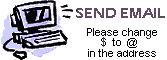W I N I O N O W
Science Trivia #1
The Contest Center
Wappingers Falls, NY 12590
 W I N I O N O W

A01:   What temperature is the same on both the Fahrenheit and Celsius scales?   [Answer]

A02:   Which liquid can dissolve wax: water, alcohol, or gasoline?   [Answer]

A03:   What is the smallest number that can be expressed two different ways as the sum of two squares? (A square means a natural number times itself, like 9 is 3x3.)   [Answer]

A04:   What is the tallest species of trees?   [Answer]

A05:   What is the thickest species of trees?   [Answer]

A06:   Lake Baikal is the deepest freshwater lake in the world. What is the temperature at the bottom?   [Answer]

A07:   What is the only liquid that can dissolve carbon?   [Answer]

A08:   In the U.S., on which side of a tree does moss usually grow?   [Answer]

A09:   What is the name of the north star?   [Answer]

A10:   What device controls current in a DC circuit?   [Answer]

A11:   What temperature is absolute zero on the Kelvin scale?   [Answer]

A12:   Which is most like a torus: football, inner tube, or cross?   [Answer]

A13:   How many prime numbers are even?   [Answer]

A14:   What is the largest living bird?   [Answer]

A15:   What is the latitude of the North Pole?   [Answer]

A16:   How much of an iceberg is underwater?   [Answer]

A17:   If the Arctic ice cap melted, how high would sea level rise?   [Answer]

A18:   What species of animal lives the longest?   [Answer]

A19:   Which snake has the longest fangs?   [Answer]

A20:   How many needles are in each tuft of a white pine?   [Answer]Send us an email to submit answers to these questions, to ask for help, or to submit new questions. Be sure to change the \$ to an @ in our email address.